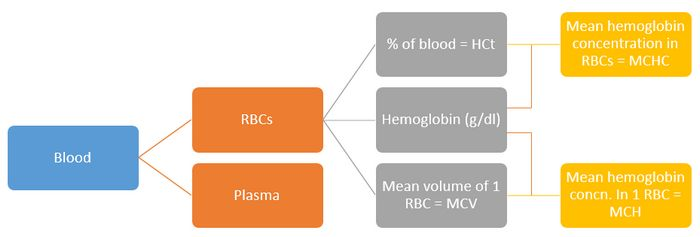# Understanding Red cell indices

## Rule of 3s

The measured hemoglobin concentration is 3 times the RBC count, and the calculated hematocrit is 3 times the Hb level. A significant deviation means artifacts in the value estimated or the RBCs are smaller or larger than the normal.

• HCt = 3 X Hb
• RBC count = Hb/3

## The units

1. Hematocrit: Percentage (%)
2. MCV: fL (femtolitres i.e. 10^-15 litres)
3. MCH: pg (picogram i.e. 10^-12 grams)
4. MCHC: g/L

1 ml = 1000 cu.mm

1 L = 1000 ml

1 L = 1000000 cu.mm

Hb (g/dL) X 10 = Hb (g/L)## Hematocrit

In simple words, hematocrit is the percentage of your blood that is made up of Red Blood Cells (RBCs).

HCt = (Total blood volume – Total Plasma volume)/Total blood volume

## Mean corposcular volume (MCV)

Mean volume of 1 RBC (Total RBC volume/Total number of erythrocytes or RBC)

MCV = (HCt X 10)/RBC count per Litre

Normal: 80-100 fL

Apply rules of 3 in the formula above: MCV = (3 X Hb X 10)/Hb/3 = 90. Hence, MCV is around 90 fL in average. Normal value is 90±10 fL.

HCt = MCV X RBC count X 0.1

## Mean corposcular hemoglobin (MCH)

Mean hemoglobin concentration in 1 RBC (Hemoglobin concentration/Total number of erythrocytes or RBC)

MCH = (Hb X 10)/RBC count per Litre

Normal: 27-33 pg

Apply rules of 3 in the formula above: MCH = (3 X RBC count X 10)/RBC count = 30. Hence, MCH is around 30 pg in average. Normal value is 30±3 pg.

## Mean corposcular hemoglobin concentration (MCHC)

Mean hemoglobin concentration in RBCs (Hemoglobin concentration/proportion of RBC)

MCHC = (Hb X 100)/HCt

Normal: 33-37 g/L

Apply rules of 3 in the formula above: (Hb X 100)/(3 X Hb) = 33. Hence, MCHC is around 33g/L in lower limit. Normal range is 35±2 g/L.

## Interconversions

MCHC =  (MCH X 100)/MCV

MCH = (MCHC X MCV)/100

MCV = (MCH X 100)/MCHC

## Red Cell Distribution Width (RDW)

Measure of anisocytosis (variability in RBC size) i.e. standard deviation of MCV

RDW = (Standard deviation of red cell volume X 100)/Mean cell volume

Normal range = 11 to 15%

## Classification of Anemia based on MCV### MCV low (Microcytic)

Normal RDW

• Thalasemmia trait
• Anemia of chronic disease
• HbH

High RDW

• Iron deficiency
• Beta-thalasemmia
• Sickle/HbC trait

### MCV normal (Normocytic)

Normal RDW

• Normal
• Anemia of chronic disease
• Hemoglobinopathies
• Hereditary spherocytosis
• Transfusion
• Chemotherapy
• CLL, CML
• Hemorrhage

High RDW

• Mixed deficiency
• Early iron or folate deficiency
• Myelofibrosis
• Sideroblastic anemia

### MCV high (Macrocytic)

Normal RDW

• Aplastic anemia
• Pre-leukemia

High RDW

• Folate deficiency
• Vitamin B12 deficiency
• Immune hemoglobin
• Cold agglutinins
• CLL

This site uses Akismet to reduce spam. Learn how your comment data is processed.